# Change Nickname

Current Nickname:
Search
v2.x
v2.x

Meta-data refers to the nodes and edges of the graphset. This chapter will introduce the operations on meta-data. As meta-data operations frequently involve Ultipa Filter, all illustrations in this chapter will be given with simple filters before the comprehensive introduction of filters coming in the next chapter.

## `insert()`

Insertion is either inserting meta-data into a graphset, or updating the existing meta-data upon recognition of duplicated _id or _o. This dual function is also called 'upsert' in Ultipa knowledge system.

### uQL Components

The uQL components of meta-data insertion:

Type Components
Command `insert()`
Parameter `nodes(<>)` or `edges(<>)`, `check_o()`, `silent()`
Return (a list of ids of the inserted meta-data)

Values of parameter:

Name Data Type Specification Description
nodes []obj (see below) (1/2) The nodes to be inserted/updated, does not coexist with `edges()`
edges []obj (see below) (2/2) The edges to be inserted/updated, does not coexist with `nodes()`
check_o / / (Optional) To check the duplication of _o for update
silent / / (Optional) To omit the list of ids of the inserted meta-data (usually in a batch insertion)

Where:

• _o will only be checked for duplication when it is provided and the `check_o()` is included in the uQL;
• once provided, _id will always be checked for duplication;
• do NOT try to check _id and _o at the same time;
• _id and _o will be generated by Ultipa system if they are not provided;
• the mapping between _id and _o of nodes is the basis for converting (_from_o, _to_o) to (_from_id, _to_id) of edges in an edge insertion;
• the data format of `nodes(<>)` and `edges(<>)` is:
``````[
{<property>:<value>, <property>:<value>, ...},
{<property>:<value>, <property>:<value>, ...},
...
]
``````

`Refer to the end of chapter Background for details on system-reserved properties.`

### Examples

#### `insert().nodes()`

Example 1: Insert a node with name = 'Ultipa' and year = 2019

``````insert().nodes( {name: "Ultipa", year: 2019} )
``````

Example 2: Insert two nodes with name = 'Ultipa' and 'database'

``````insert().nodes( [ {name: "graph"}, {name: "database"} ] )
``````

Example 3: Insert a node with name = 'metadata' and _o = 'uuid00001'

``````insert().nodes( {_o: "uuid00001", type: "metadata"} )
``````

Example 4: Insert a node with name = 'path' and _o = 'uuid00001', check _o for duplication

``````insert().nodes( {_o: "uuid00001", type: "path"} ).check_o()
``````

`When _o is provided, the check_o() can be omitted to accelerate the insert operation, but only with the guarantee that the values of declared _o are unique.`

#### `insert().edges()`

Example 1: Insert an edge with _from_id = 1 and _to_id = 2, and type = 'college'

``````insert()
.edges( {_from_id: 1, _to_id: 2, type: "college"} )
``````

Example 2: Insert an edge with _from_o = 'uuid00001' and _to_o = 'uuid00002'

``````insert()
.edges( {_from_o: "uuid00001", _to_o: "uuid00002"} )
``````

Example 3: Insert an edge with _id = 10, _from_id = 1 and _to_id = 4, and use silent mode

``````insert()
.edges( {_from_id: 1, _to_id: 2, _id: 10} )
.silent()
``````

`The nodes represented by the values of _from_id, _to_id, _from_o or _to_o must already exist in the graphset before inserting edges, to guarantee a successful edge insert operation.`

## `update()`

uQL supports modifying property values in real-time, against specific meta-data that satisfy certain filtering criteria.

### uQL Components

The uQL components of meta-data update:

Type Components
Command `update()`
Parameter `nodes(<>)` or `edges(<>)`, `set(<>)`
Return (operational status)

Values of parameter:

Name Data Type Specification Description
nodes obj Ultipa filter (1/2) The nodes to be updated, does not coexist with `edges()`
edges obj Ultipa filter (2/2) The edges to be updated, does not coexist with `nodes()`
set obj (see below) The new values of properties for update

Where:

• _id and _o cannot be updated with this command;
• all the meta-data satisfying the criteria will be updated with the same set of `property:value` pairs defined in `set(<>)` with format:
``````{ <property>:<value>, <property>:<value>, ... }
``````

### Examples

#### `update().nodes()`

Example 1: Update node id = 12, set its name to 'Ultipa'

``````update()
.nodes(12)
.set( {name: "Ultipa"} )
``````

Example 2: Update a set of nodes with ids = [12,23,30] to set their type to 'Company'

``````update()
.nodes([12, 23, 30])
.set( {type: "Company"} )
``````

Example 3: Update nodes where age > 18 to make their type to "adult"

``````update()
.nodes( {age: {\$gt: 18} } )
``````

Example 4: Update the node that has original ID = 'Ricky_is_here_world', and set name to 'Ultipa v3.1'

``````update()
.nodes( {_o: "Ricky_is_here_world"} )
.set( {name: "Ultipa v3.1"} )
``````

#### `update().edges()`

Example 1: Update edges that share the _from_id = 12 to have their name = "Like"

``````update()
.edges( {_from_id: 12} )
.set( {name: "Like"} )
``````

See comparison on edges before and after the update operation: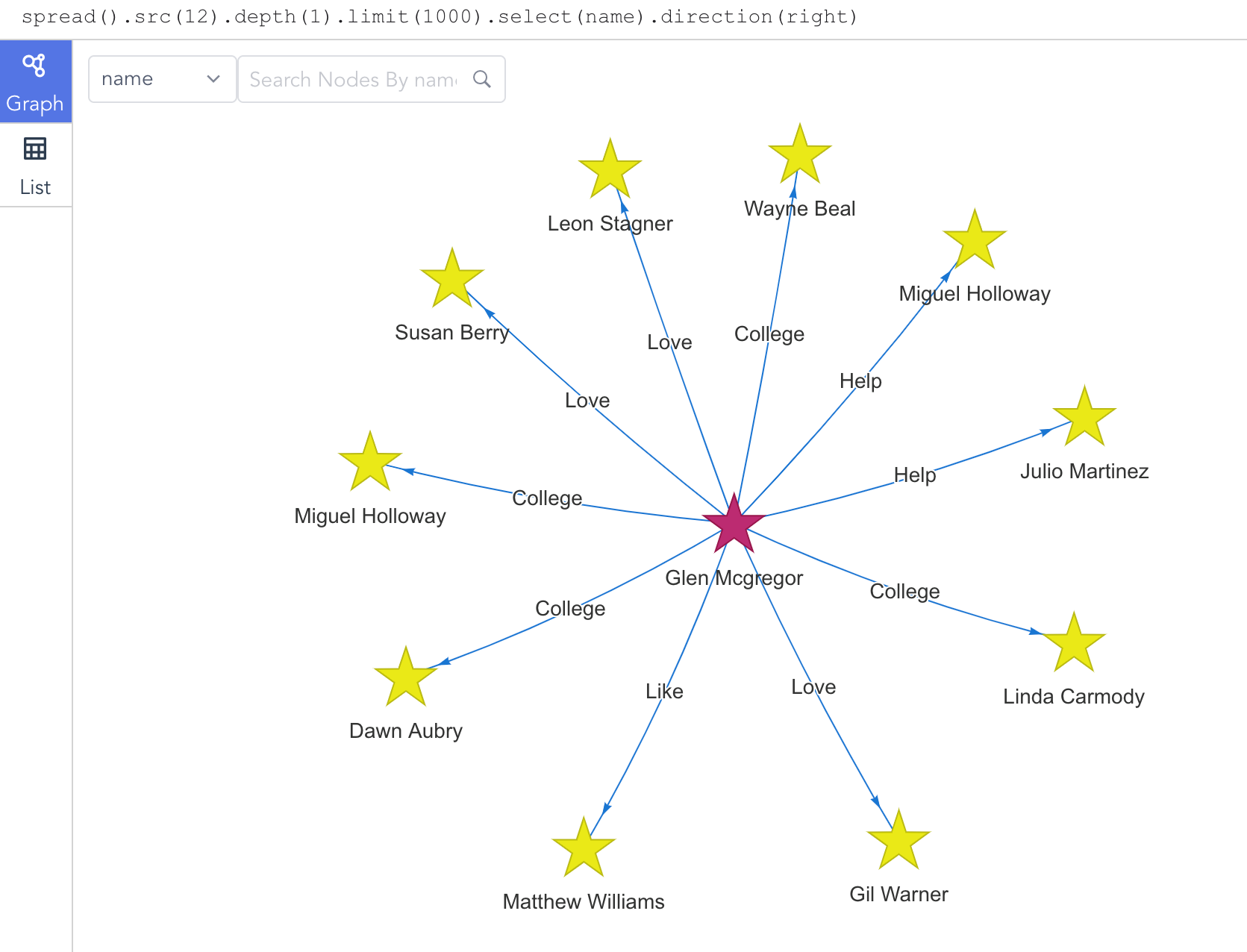Figure: Update Edge Before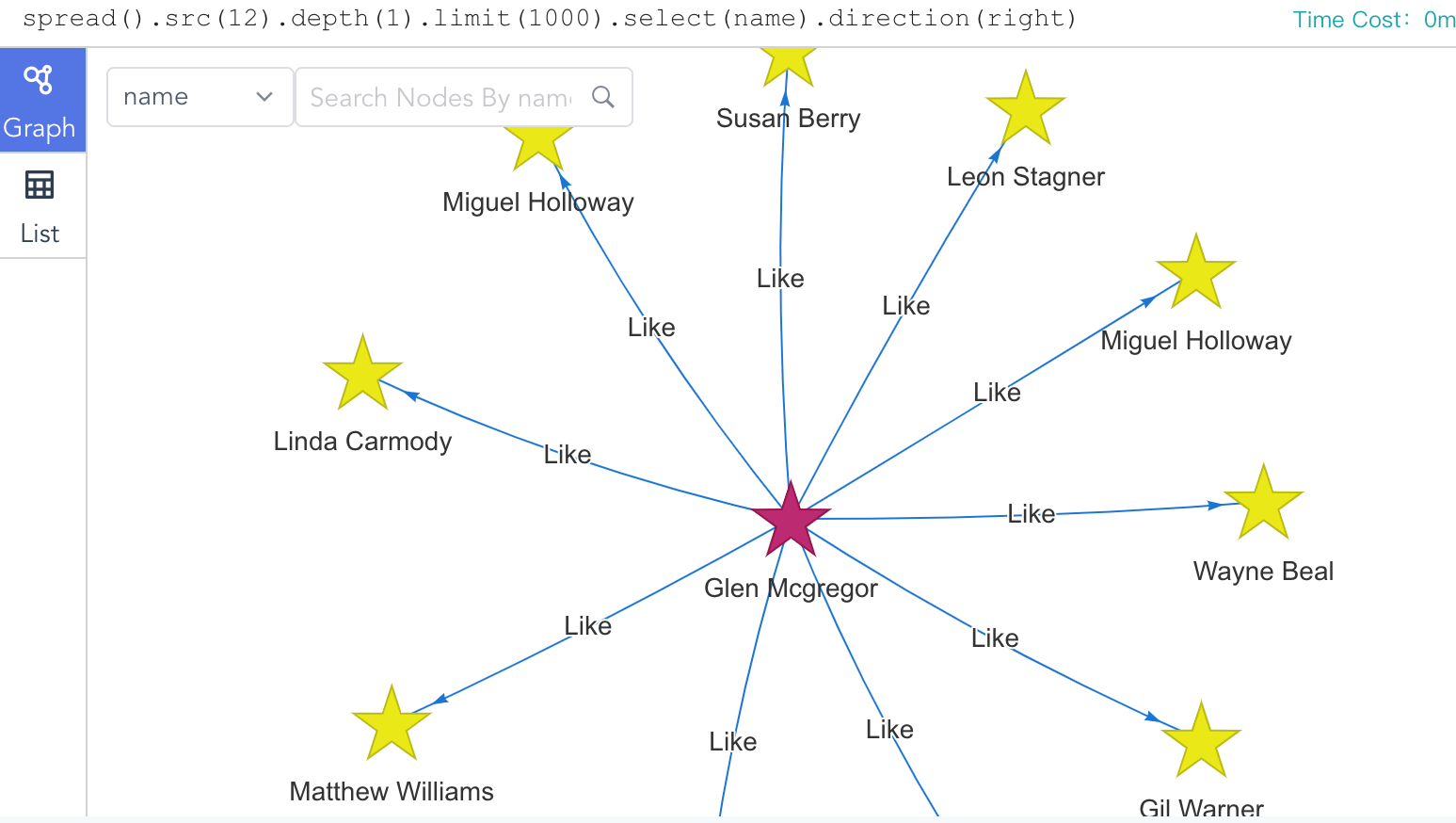Figure: Update Edge After

## `delete()`

Deletion can be done against nodes or edges that are no longer needed. Before executing deletion, especially with sophisticated filters, make sure you know exactly what you are doing, because these operations are NOT reversible.

### uQL Components

The uQL components of meta-data deletion:

Type Components
Command `delete()`
Parameter `nodes(<>)` or `edges(<>)`
Return (operational status)

Values of parameter:

Name Data Type Specification Description
nodes obj Ultipa filter (1/2) The nodes to be deleted, does not coexist with `edges()`
edges obj Ultipa filter (2/2) The edges to be deleted, does not coexist with `nodes()`

### Examples

#### `delete().nodes()`

Example 1: Delete node id = 12

``````delete().nodes(12)
``````

Example 2: Delete nodes where type = expire or age >= 60

``````delete().nodes( {\$or: [ {type: "expire"}, {age: {\$gte: 60} } ] } )
``````

#### `delete().edges()`

Example 1: Delete edge id = 1200

``````delete().edges(1200)
``````

Example 2: Delete edges that with _from_id = 122

``````delete().edges( {_from_id: 122} )
``````

See comparison on edges before and after the delete operation in Example 2: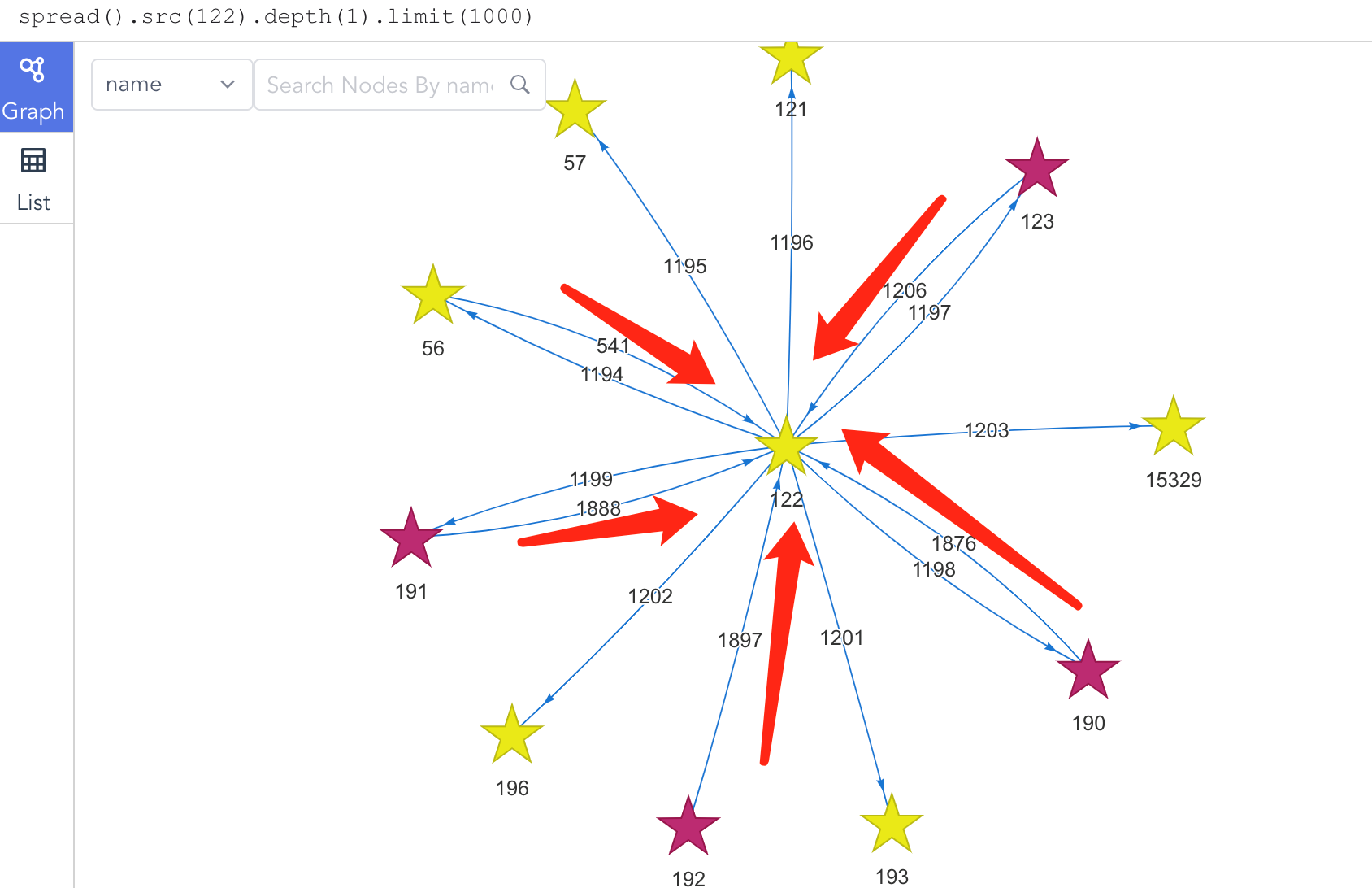Figure: Delete Edge Before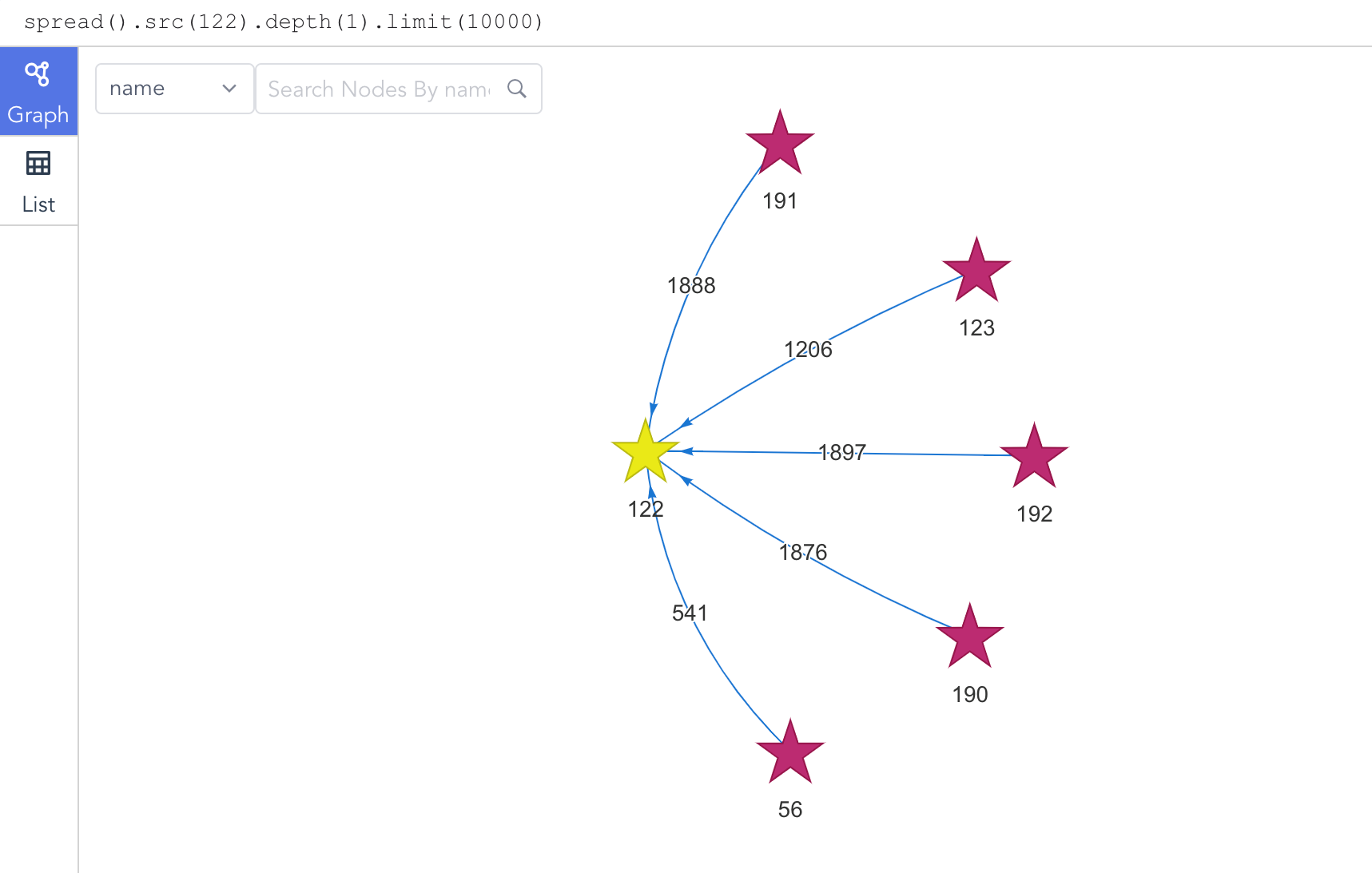Figure: Delete Edge After

## `truncate()`

Truncate is the delete operation done against all the meta-data of a graphset at one time. This command is also NOT reversible.

The uQL components to clear all meta-data in the graphset:

Type Components
Command `truncate(<>)`
Parameter `graph(<>)`
Return (operational status)

Values of command and parameter:

Name Data Type Specification Description
truncate string 'nodes' or 'edges' or / To delete all the nodes, or edges, or both from the graphset
graph string graphset name The name of the graphset

Example 1: Erase all the nodes from graphset 'default':

``````truncate(nodes).graph("default")
``````

Example 2: Erase all the edges from graphset 'default':

``````truncate(edges).graph("default")
``````

Example 3: Erase all the meta-data from graphset 'default':

``````truncate().graph("default")
``````

## `compact()`

Compact is to clear useless, redundant data in the graphset, which is comparable to the defragmentation in a Windows system.

The uQL components to compact the graphset:

Type Components
Command `compact()`
Parameter `graph(<>)`
Return (operational status)

Example: Compact garphset 'default'

``````compact().graph("default")
``````

## `find()`

Queries against meta-data are comparable to traditional database queries; given certain filtering rules, relevant meta-data will be returned.

### uQL Components

The uQL components of meta-data query:

Type Components
Command `find()`
Parameter `nodes(<>)` or `edges(<>)`, `limit(<>)`
Return `select(<>)`

Values of parameter and return:

Name Data Type Specification Description
nodes obj Ultipa filter (1/2) To find nodes, does not coexist with `edges()`
edges obj Ultipa filter (2/2) To find edges, does not coexist with `nodes()`
limit int >0; -1 (Optional) The maximum number of nodes or edges to return; -1: return all the results
select []string comma (,) separated; * (Optional) A list of properties to return; *: return all the properties

Where:

• when `limit()` is not included in the uQL, it's considered as `limit(3)`;
• _id, _from_id and _to_id are the default properties to be returned and need not to be declared in `select()`.

### Examples

#### `find().nodes()`

Example 1: Find the node whose id is 3 and return its original id

``````find()
.nodes(3)
.select(_o)
``````

Running Example 1 within Ultipa-Manager will get this: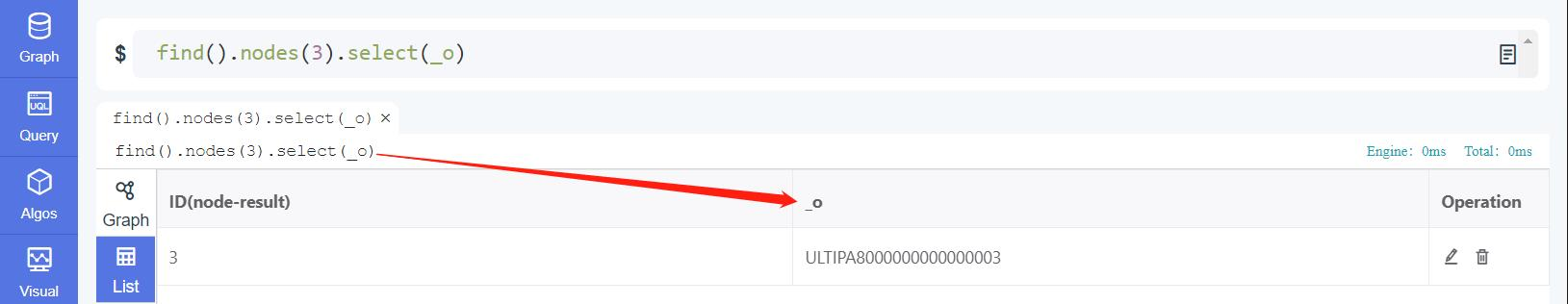Figure: Find Node and Show Original ID

Example 2: Find nodes whose ids are 12, 21 and 32, and return all their properties

``````find()
.nodes([12,21,32])
.select(*)
``````

Example 3: Find nodes with 'year' between 1998 and 2005, return their 'industry', limit up to 5 results

``````find()
.nodes( {year: {\$bt: [1998, 2005]} } )
.limit(5).select(industry)
``````

Running Example 3 within Ultipa-Manager will get this: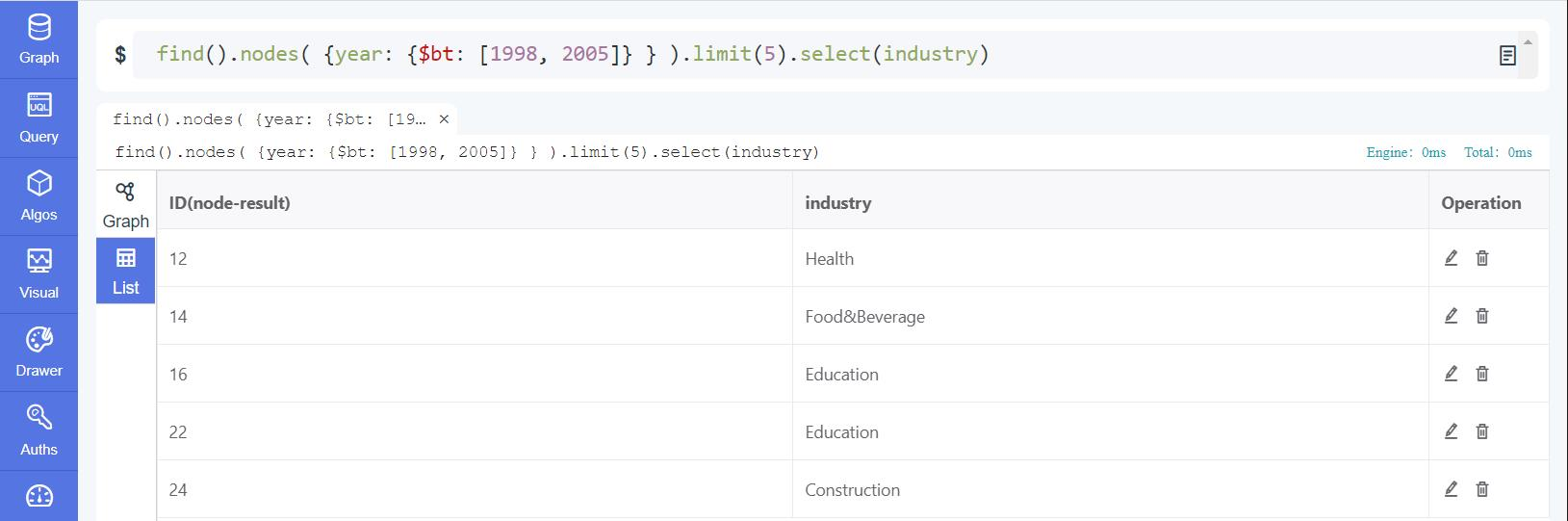Figure: Find Nodes with Ultipa Manager

#### `find().edges()`

Example 1: Find the edge whose id is 12

``````find()
.edges(12)
``````

Example 2: Find edges that start from node (_id = 12), return their 'type', limit up to 3 results

``````find()
.edges( {_from_id: 12} )
.limit(3).select(type)
``````

Running Example 2 within Ultipa-Manager will get this: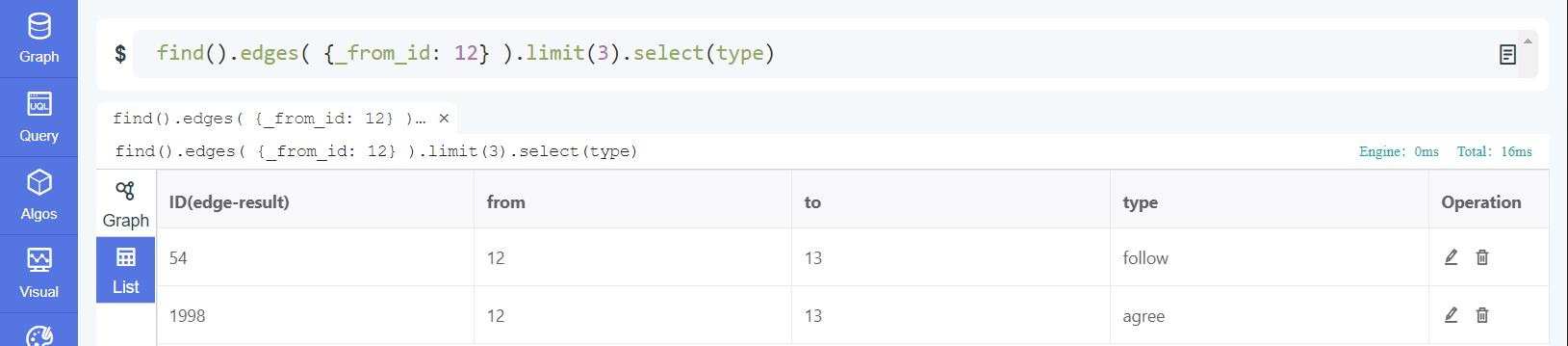Figure: Find Edges with Ultipa Manager 1

Example 3: Find edges whose types are either 'review' or 'response', return their type and timestamp, limit up to 7 edges

``````find()
.edges( {type: {\$in: ["review", "response"]} } )
.limit(7).select(type, timestamp)
``````

Running Example 3 within Ultipa-Manager will get this: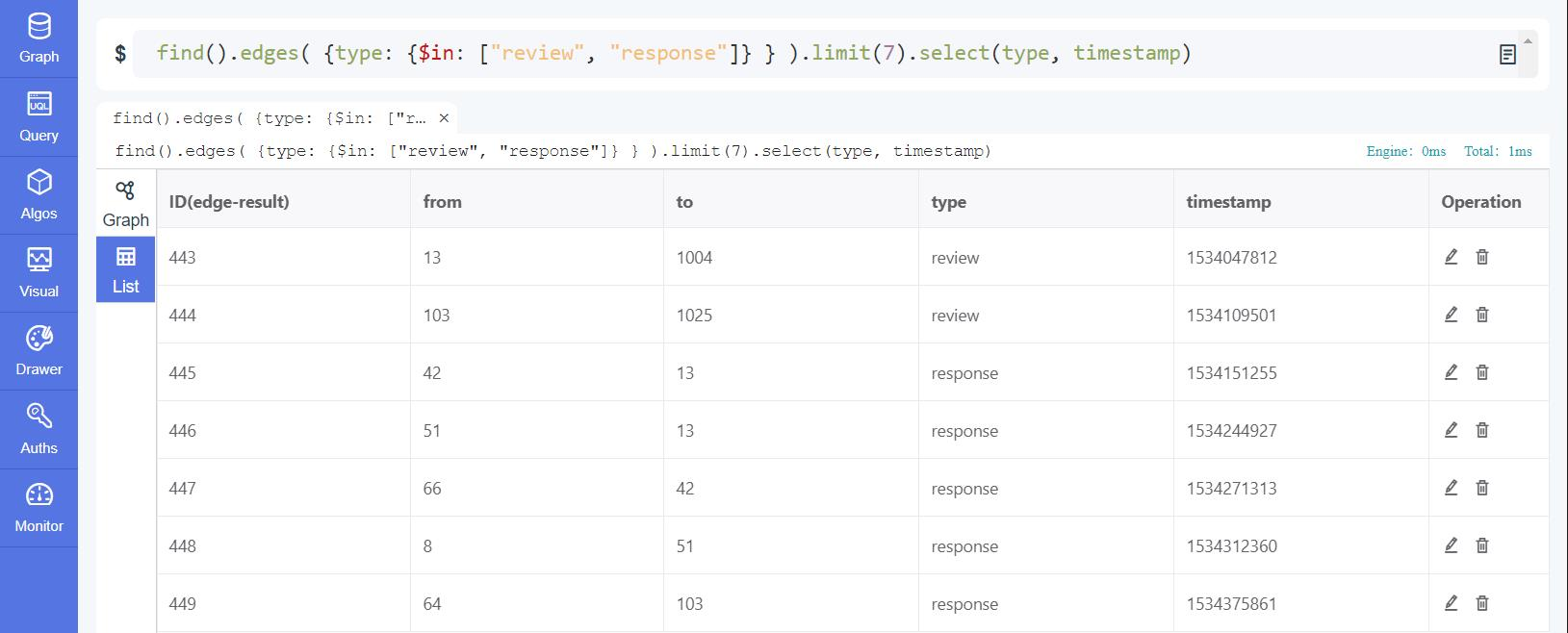Figure: Find Edges with Ultipa Manager 2